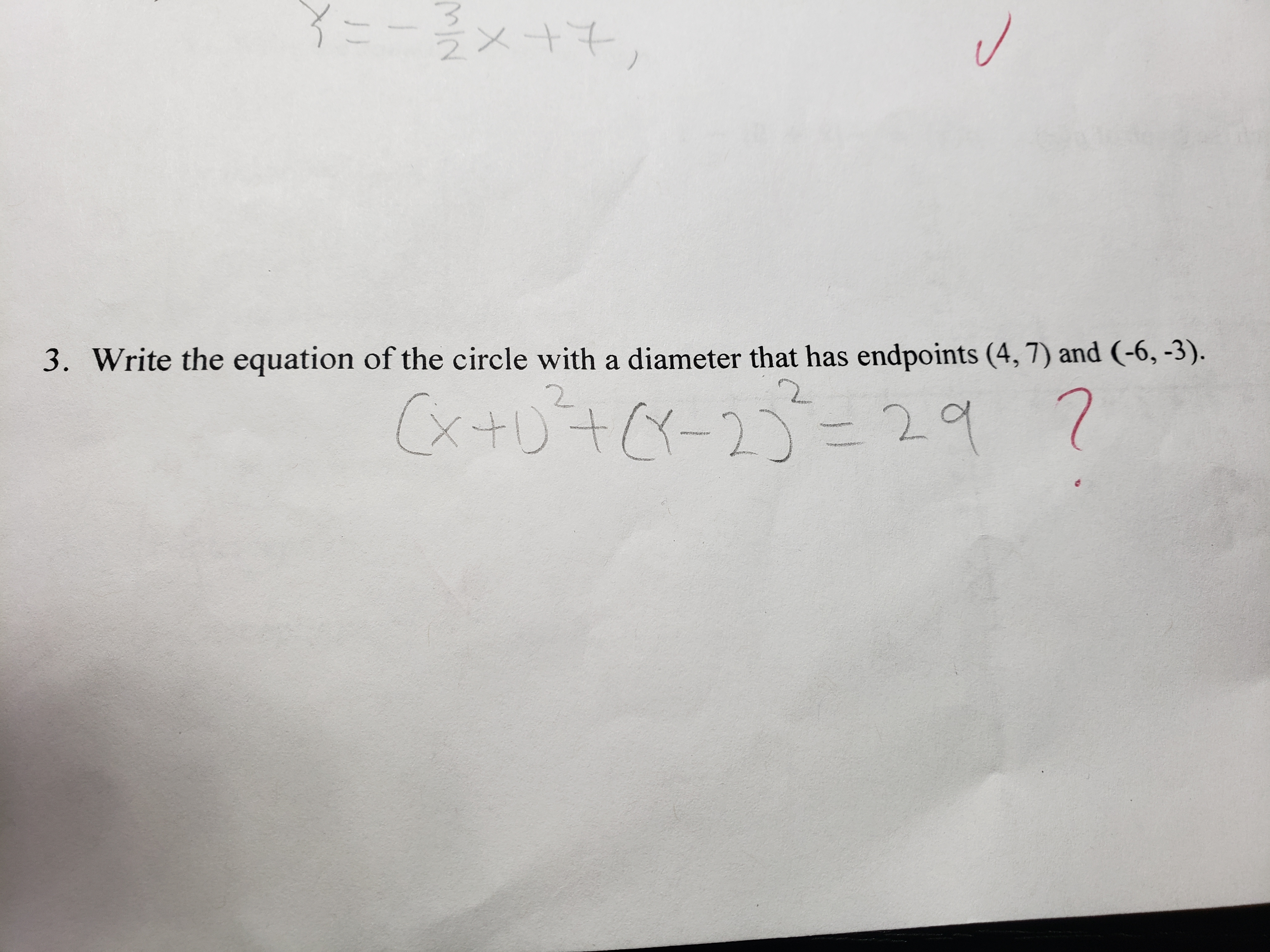3. Write the equation of the circle with a diameter that has endpoints (4,7) and (-6,-3),22.

Questionhelp_outlineImage Transcriptionclose3. Write the equation of the circle with a diameter that has endpoints (4,7) and (-6,-3), 2 2. fullscreen
Step 1

To determine the equation the circle with the given properties

Step 2

Here is a rough sketch of the problem statement. We need to find the coordinates of the center C and the radius CP=CQ

Step 3

The center C and the ra...

Want to see the full answer?

See Solution

Want to see this answer and more?

Our solutions are written by experts, many with advanced degrees, and available 24/7

See Solution
Tagged in

Algebra